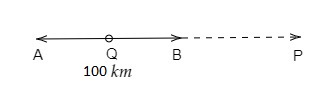# Places A and B are 100 km apart on a highway. One car starts from A and another from B at the same time. If the cars travel in the same direction at different speeds, they meet in 5 hours. If they travel towards each other, they meet in 1 hour. What are the speeds of two cars?

Given:

Places A and B are 100 km apart on a highway. One car starts from A and another from B at the same time. If the cars travel in the same direction at different speeds, they meet in 5 hours. If they travel towards each other, they meet in 1 hour.

To do:

We have to find the speed of the two cars.

Solution:

We know that,

Distance$=$ Speed $\times$ Time.

Distance between the two places A and B $= 100\ km$.

Let the speed of the first car starting from A be $x\ km/hr$ and the speed of the second car starting from B be $y\ km/hr$.Let the cars meet at point P when they are moving in the same direction and at point Q when they are moving in the opposite direction.

When they travel in the same direction, they meet in 5 hours.

Distance travelled by the first car in 5 hours $AP= 5\times x\ km=5x\ km$.

Distance travelled by the second car in 5 hours $BP= 5\times y\ km=5y\ km$.

$AP-BP=100$

$5x-5y=100$

$5(x-y)=5\times20$

$x-y=20$.....(i)

When they travel in the opposite direction, they meet in 1 hour.

Distance travelled by the first car in 1 hour $AQ= 1\times x\ km=x\ km$.

Distance travelled by the second car in 1 hour $BQ= 1\times y\ km=y\ km$.

$AQ+BQ=AB$

$x + y = 100$….(ii)

Adding equations (i) and (ii), we get,

$x-y+x+y=20+100$

$2x = 120$

$x = \frac{120}{2}$

$x=60$

Substituting $x=60$ in equation (ii), we get,

$60+y=100$

$y = 100-60$

$y = 40$

Therefore, the speed of the first car is $60\ km/hr$ and the speed of the second car is $40\ km/hr$.